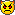## Problem J2: Mod Inverse

In many cryptographic applications the Modular Inverse is a key operation. This question involves finding the modular inverse of a number.

Given 0 < x < m, where x and m are integers, the modular inverse of x is the unique integer n, 0 < n < m, such that the remainder upon dividing x × n by m is 1.

For example, 4 x 13 = 52 = 17 x 3 + 1, so the remainder when 52 is divided by 17 is 1, and thus 13 is the inverse of 4 modulo 17.

You are to write a program which accepts as input the two integers x and m, and outputs either the modular inverse n, or the statement "No such integer exists." if there is no such integer n.

You may assume that m ≤ 100.

`417`

`13`

`610`

### Sample Output 2

`No such integer exists.`

Point Value: 3
Time Limit: 2.00s
Memory Limit: 16M

Problem Types: [Show]

Languages Allowed:
C++03, PAS, C, HASK, ASM, RUBY, PYTH2, JAVA, PHP, SCM, CAML, PERL, C#, C++11, PYTH3

• (0/4)
I dont understand what a modular inverse is.
The question didnt explain.
It only gave a example.
Can someone explain this for me?

• (3/0)
the modular inverse of x is the unique integer n, 0 < n < m, such that the remainder upon dividing x × n by m is 1.

• (0/2)
it explained, look carefully.• (0/1)
^ OMG SATAN!!! >>OMG

• (1/0)
by the way, wtf stands for well thats fantastic

• (1/1)
>>SATAN AGAIN :O:O:O